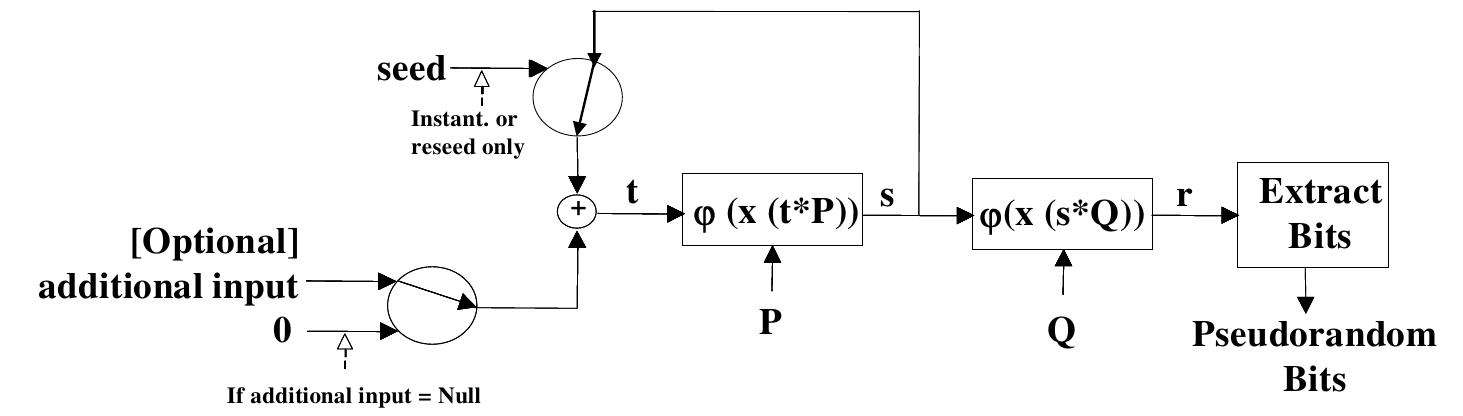# Dual EC DRBG

## Description of Dual EC

This page explains how Dual EC is defined. The authoritative description is in NIST Special Publication 800-90.

### Background on elliptic curve cryptography

Dual EC uses elliptic curves of the form E: y2=x3+ax+b, defined over a finite field Fp, where p is a prime. Addition, subtraction, and multiplication in Fp are addition, subtraction, and multiplication of integers modulo p: one brings each result into the range {0,1,...,p−1} by adding or subtracting any necessary multiple of p. Any two points on an elliptic curve can be added using certain addition laws; note that there are some special cases, e.g. adding a point to itself (=doubling), which require somewhat different formulas, but in any case the addition operation leads to a further point on the curve. For P=(x,y) we have −P=(x,−y). Adding together k copies of P is referred to as scalar multiplication (k is the scalar here) and produces a result denoted kP. The point P is referred to as the base point. This operation can be done more efficiently using the double-and-add method. Note that the x-coordinate of kP is the same as the x-coordinate of k(−P)=−(kP). While computing kP takes log2(k) steps, finding k given Q=kP takes time exponential in the order of P for well-chosen elliptic curves. The value k is called the discrete logarithm of Q to the base of P. For more details see, e.g., the Wikipedia entry on ECC.

There are three versions of Dual EC, using the three curves standardized in NSA Suite B for ECC applications. Each curve is specified by a prime p, an elliptic curve E over Fp, and a point P on E; for each curve the Dual EC standard also specifies a second point Q on E. Each parameter set corresponds to a different security level. The most widely used curve among the Suite B curves is P-256, with p = 2256 − 2224 + 2192 + 296 − 1 and E: y2 = x3−3x+41058363725152142129326129780047268409114441015993725554835256314039467401291.

### How Dual EC works

Dual EC was published with the following graphic [page 68 of SP800-90-2012] depicting the Dual EC data flow.In this description P and Q are the two standard Dual EC points on an elliptic curve. Scalar multiplication (see above) is denoted with an extra *, i.e. t*P instead of tP, x(R) denotes the x-coordinate of R. For a point R over Fp the x-coordinate is an element of Fp. The map φ takes an element of Fp, considers it as an integer in {0,1,...,p−1}, computes the bit representation of this integer and then truncates, removing the least significant b bits, for some fixed b. The result might be seen as a bit string or as an integer, depending on context. For Dual EC instantiated with P-256 the most significant b=16 bits are truncated.

Here are the details of how Dual EC produces an output string of any specified length. The simplest case is that the specified length fits into one block of φ output and there is no additional input. In this case the current seed s is used to produce a new seed s (used for the next Dual EC call) and an output string as follows:

1. Replace s with x(sP): i.e., compute sP and take the x-coordinate to obtain a new s.
2. Compute r=φ(x(sQ)).
3. Output the specified number of bits of r.

This is indicated in the diagram by the horizontal arrows to "Extract Bits". If the specified length is longer, but there is still no additional input, then the same steps are iterated:

1. Repeat the next steps until the output has the required length:
1. Replace s with x(sP).
2. Compute r=φ(x(sQ)).
3. Append r to the output.

If there is additional input then the seed is updated at the beginning of each call:

1. Replace s with s⊕Hash(input) if there is additional input.
2. Repeat the next steps until the output has the required length:
1. Replace s with x(sP).
2. Compute r=φ(x(sQ)).
3. Append r to the output.

What we have described so far is the original version of Dual EC. The Dual EC standard was updated in 2007 to include a further update step [page 74 of SP800-90-2012] although the diagram in the standard was not modified to show this step:

1. Replace s with s⊕Hash(input) if there is additional input.
2. Repeat the next steps until the output has the required length:
1. Replace s with x(sP).
2. Compute r=φ(x(sQ)).
3. Append r to the output.
3. Replace s with x(sP).

This modification to the standard affects the exploitability of Dual EC.